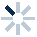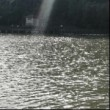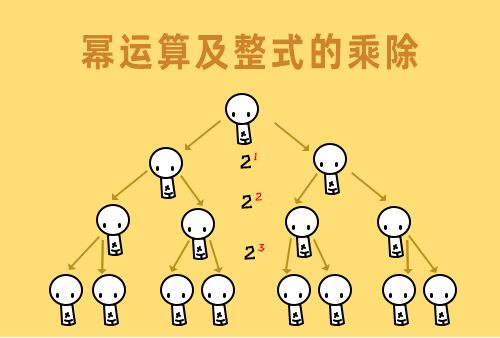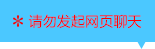| | |

||
APP端下载AndroidiPhone
|
￥48

|
2674人点赞
279455人已学习
|

3天无理由退款

3天无理由退款：退款将以超级币形式退至您的超级课堂学习账户，便于您重新选购其他课程。恶意退款将被冻结账号。

• 1、反比例函数的概念，当两个变量的乘积是一个固定的，不为$0$的常数时，它们就是反比例的关系。
2、 反比例函数的解析式是：$y=\dfrac{k}{x}$（$k$是常数，且$k\neq 0$），$k$也叫做比例系数。
3、 反比例函数必须满足的三点：(1)、$k$是常数且$k$不为零。(2)、自变量$x$的指数是$-1$。(3)、解析式中除了比例系数$k$外没有其他常数。同时它的定义域是$x\neq 0$。
4、 求反比例函数解析式用待定系数法。注意多个函数在同一个式子中出现时，要用不同的字母来表示系数$k$。
• 1、两支双曲线，无限接近$x$轴和$y$轴，但和坐标轴没有交点。
2、 反比例函数的图象既是中心对称图形，又是轴对称图形。
3、 对称中心是原点，当$k＞0$时对称轴是直线$y=x$。当$k$大于$0$时，图象位于一、三象限。当$k$小于$0$时对称轴是直线$y=-x$。
4、 $k$对图像的影响：当$k$大于$0$时，图象位于一、三象限；当$k$小于$0$时，图象位于二、四象限。$\left | k \right |$决定了图象距离坐标轴的远近，$\left | k \right |$越大，图象离坐标轴越远。
• 1、反比例函数的增减性：当$k＞0$时，在每个象限内，$y$随$x$的增大而减小；当$k＜0$时，在每个象限内，$y$随$x$的增大而增大。凡是不强调“每一支曲线”的说法都是错的。
2、 通过$x$的大小关系，判断$y$的大小关系时，如果几个$x$在$0$的同侧，也就是在同一支曲线上，那就只需根据$k$的正负判断$y$的大小；如果几个$x$在$0$的两侧，就需要你画图象，根据点的高低来判断$y$的大小。
• 1、我们学习了根据$x$的范围，求分式的范围。像$\dfrac{k}{Ax+B}$（$A$、$B$、$k$是常数，且$A$和$k$都不为$0$）这种形式，只要把分母看成一个整体就OK了。记住，你一定要看图说话。
2、 跟别的函数不同，反比例函数存在两支，在原点处都会有一个逆袭，这就是引发血案的根源。通过画图分析解决这个难点后，反比例函数增减性问题迎刃而解啦。
• 1、正比例和反比例函数图像的位置关系，关键就是判断$k_{1}$$k_{2}$是否同号，当它们相交时，两个交点关于原点对称。
• 1、一次函数和反比例函数图像的位置关系，有相离、相切、相交三种，它们分别有$0$个、一个、两个交点。判断时需要联立方程组，确定一元二次方程根的情况，看看判别式的正负。
2、 其实不论图像的形态，涉及到位置关系时，我们的策略都是完全一样的，联立解析式。这样就把几何问题，成功的转化为计算就能解决的代数问题，再次体现了数形结合的神奇简约。
• 1、反比例函数中比例系数的几何意义，它决定了双曲线矩形的面积$\left | k \right |$。
2、 同样也会有两个双曲线三角形，面积都固定为$\left | k \right |$的一半。
• 1、我们看了一场双曲线上演的百变大咖秀。各种变化，其实本质都是一样的，只要点在双曲线上，它就会遵循上节课的规律，所以以后见到了梯形甚至怪异的多边形，也不要害怕哦。
• 1、​教你利用设点法巧妙解决单条双曲线内嵌几何图形的问题。不论多么复杂的几何图形，只要找到图形中的关键点，设出点的坐标，再利用几何知识找到坐标的内在联系，同时利用解析式加以限定。就可以通过列方程解出所设点的坐标啦。
2、 同时记住一个小结论，同一条双曲线上，如果$A$的横坐标是$B$的$n$倍，那么$A$的纵坐标就是$B$的$\dfrac{1}{n}$。
• 1、学习利用设点法，巧妙解决多条双曲线内嵌几何图形的问题。
2、 不论多么复杂的几何图形，只要找到图形中的关键点，设出点的坐标，再利用几何知识找到坐标的内在联系，同时利用解析式加以限定。就可以通过列方程解出所设点的坐标啦。
• 反比例函数综合练习

• 1超级学员654885
• 2超级学员735712
• 3此间星尘
• 4小Eva
• 5Enthu
• 6唯爱我段
• 7小飞棒来拉
• 8超级学员3779939
• 9何博文
• 10超级学员4150538212465人在学
￥ 88 ￥ 88262322人在学
￥ 12 ￥ 12211139人在学
￥ 88 ￥ 88211427人在学
￥ 63 ￥ 63

• 0
• 点击分享有好礼
••app端下载关注微信号